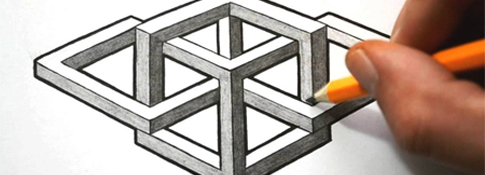##### Geometric Shapes #4###### Source: Listen In English

A simple drawing of geometric shapes including squares, circles, rectangles, triangles, vertical lines, horizonatal lines and diagonal lines.###Directions

1. REVIEW the vocabulary.
2. LISTEN to the audio above.
3. DRAW the picture (on a piece of paper).

###Vocabulary

If you don't know any of the words below, check the Illustrated Dictionary.

• a circle
• a square
• a triangle
• a line
• a star
• horizontal
• vertical
• upside down
• right-side up

###Grammar (articles)

Directions: 1) Choose the determiner that you think best fits the sentence. 2) Listen again and check your answers.

• This is (a) picture of (Ø) two triangles and two circles – one in (Ø) each corner of (the) page.
• In (the) top left corner, there is (a) medium-sized, upside down triangle.
• In (the) middle of (the) triangle, there is (a) vertical line.
• Above (the) triangle, there is (a) horizontal line.
• In (the) top right corner of (the) page, there is (a) medium-sized circle.
• Inside (the) circle, there is (a) horizontal line.
• In (the) bottom left corner of (the) page, there is (a) right-side up triangle.
• It is (the) same size as (the) first triangle.
• In (the) middle of (the) triangle, there is (a) vertical line.
• Below (the) triangle, there is (a) horizontal line
• In (the) bottom right corner of (the) page, there is (Ø) another circle.
• It’s (a) little bit bigger than (the) first circle.
• In (the) middle of (the) circle, there is (a) horizontal line.
• On (the) right side of (the) circle, there is (a) small star.

###Script

This is a picture of two triangles and two circles – one in each corner of the page.

In the top left corner, there is a medium-sized, upside down triangle.

In the middle of the triangle, there is a vertical line.

Above the triangle, there is a horizontal line

In the top right corner of the page, there is a medium-sized circle.

Inside the circle, there is a horizontal line.

In the bottom left corner of the page, there is a right side up triangle.

It is the same size as the first triangle.

In the middle of the triangle, there is a vertical line.

Below the triangle, there is a horizontal line

In the bottom right corner of the page, there is another circle.

It’s a little bit bigger than the first circle.

In the middle of the circle, there is a horizontal line.

On the right side of the circle, there is a small star.